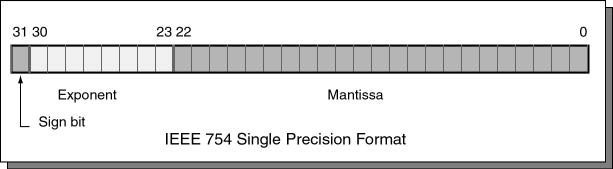It is not represented. (Remember, the biased exponent 0000 0000 is used for the value zero).

# Float FormulaHere is a formula that summarizes the past several pages. In it, `s` is the sign bit (0 or 1), `M` is the mantissa (000...000 to 111...111) and `E` is the biased exponent.

```value = (-1)s × 1.M × 2E-127
```

To convert a paper-and-pencil number into IEEE floating point, fill in each piece of the picture. Let us represent 1.0 as a 32-bit IEEE 754 float.

### QUESTION 17:

• What is the sign bit of 1.0?
• What is the mantissa of 1.0? (Hint: remember the assumed "1." preceding the mantissa)
• What is the actual exponent of 1.0?
• What is the biased exponent of 1.0? (Hint: add the actual exponent to 127)